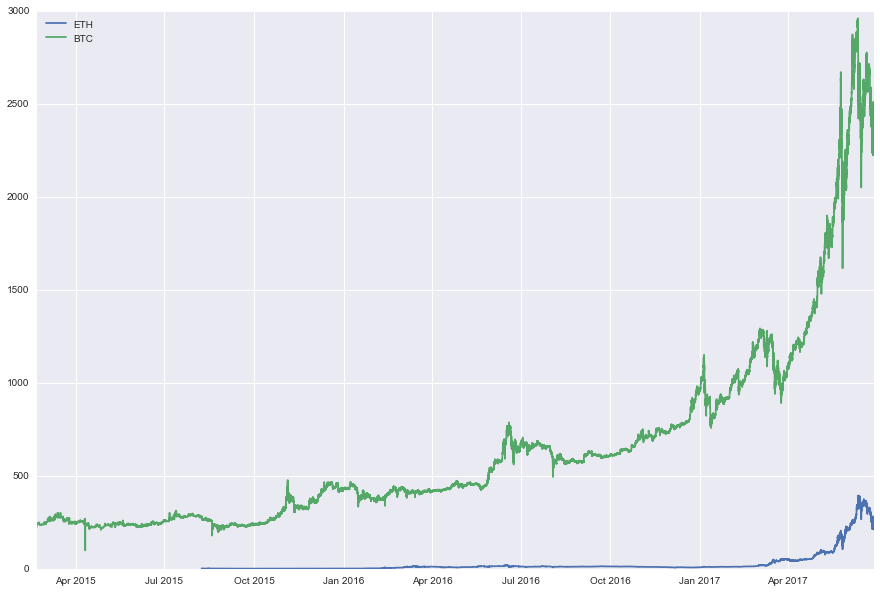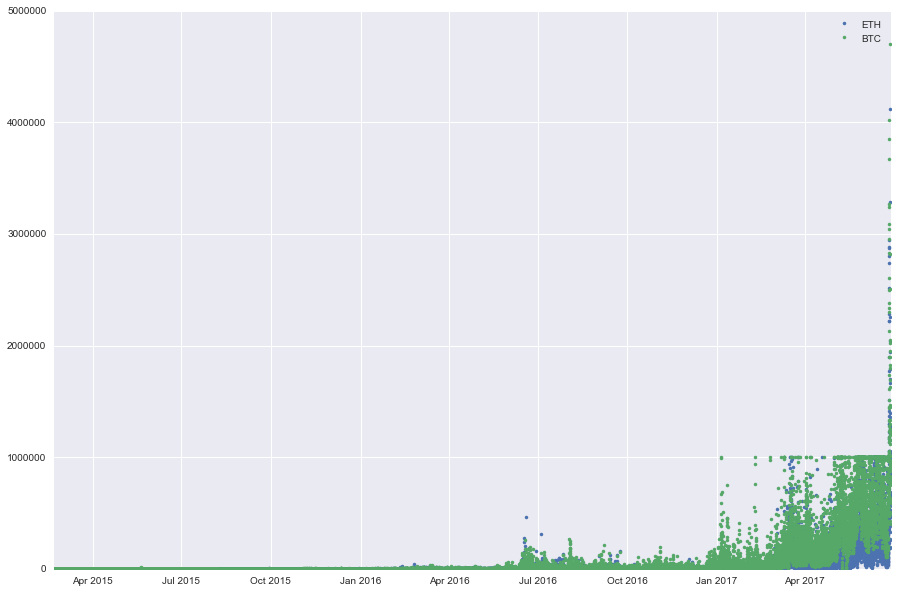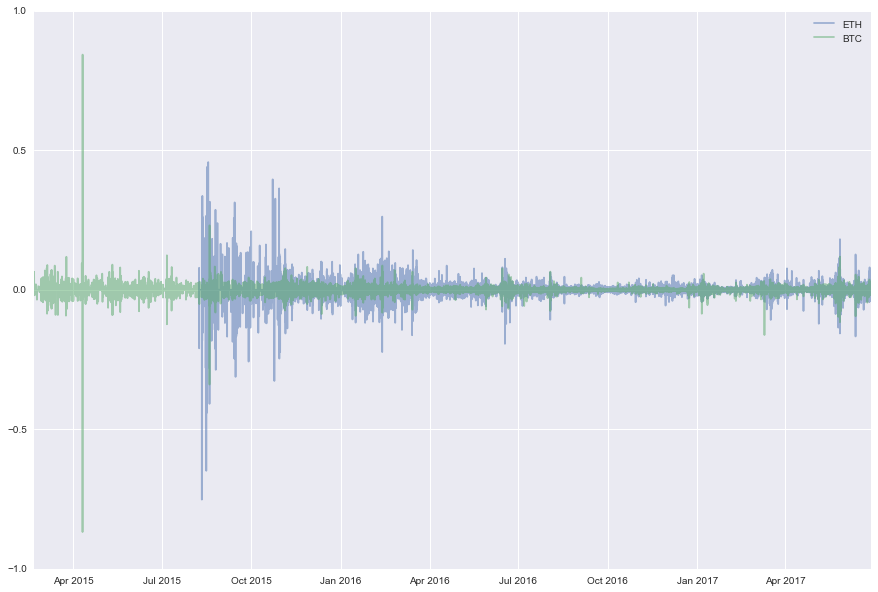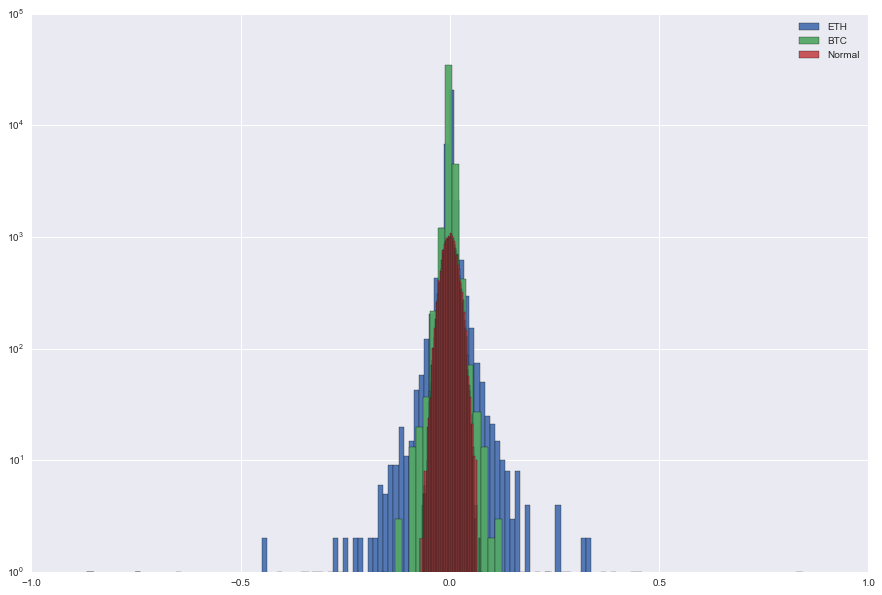For the last few days, ETH has lost 1/3 of its value with -20% several days in a row. We update the initial study with up-to-date data to take into account this recent drawback. We add a comment on the skewness of the returns distribution at the end.

``````import numpy as np
import scipy
import pandas as pd
import matplotlib.pyplot as plt
%matplotlib inline
import seaborn as sns
``````

We load historical data for ETH/USD and BTC/USD prices into pandas dataframe:

``````histo_BTC = pd.read_csv('BTCUSDT_1.csv')
``````

We convert the time in seconds of the ‘date’ column into dates:

``````histo_ETH['date'] = pd.to_datetime(histo_ETH['date'],unit='s')
histo_BTC['date'] = pd.to_datetime(histo_BTC['date'],unit='s')
``````

We take a look at the dataframes, we display the tail and head of the dataframe for ETH/USD prices:

``````histo_ETH.tail()
``````
date high low open close volume quoteVolume weightedAverage
33122 2017-06-28 07:00:00 273.340000 266.5 266.500000 271.000000 787049.632584 2911.991623 270.278811
33123 2017-06-28 07:30:00 276.280002 270.0 270.000070 276.280002 460955.337537 1680.342777 274.322206
33124 2017-06-28 08:00:00 280.280070 275.0 276.280002 280.000000 586820.959374 2114.449571 277.528945
33125 2017-06-28 08:30:00 280.279000 268.6 280.100000 276.525969 833818.197678 3043.753814 273.944034
33126 2017-06-28 09:00:00 280.754655 276.4 276.525969 279.144106 515923.390002 1846.289433 279.437980
``````histo_ETH.head()
``````
date high low open close volume quoteVolume weightedAverage
0 2015-08-08 06:00:00 1.85 1.61 1.65 1.85 90.024655 53.0404 1.697286
1 2015-08-08 06:30:00 1.85 1.71 1.85 1.71 20.686119 12.0898 1.711040
2 2015-08-08 07:00:00 1.85 0.50 1.75 1.85 33.717419 18.8862 1.785290
3 2015-08-08 07:30:00 1.85 1.85 1.85 1.85 0.000000 0.0000 1.850000
4 2015-08-08 08:00:00 1.85 1.85 1.85 1.85 0.000000 0.0000 1.850000

Below, we display their price time series:

``````plt.figure(figsize=(15,10))
plt.plot(histo_ETH['date'],histo_ETH['close'])
plt.plot(histo_BTC['date'],histo_BTC['close'])
plt.legend(['ETH','BTC'],loc=0)
plt.show()
``````And the volumes traded for the two crypto/USD rates:

``````plt.figure(figsize=(15,10))
plt.plot(histo_ETH['date'],histo_ETH['volume'],'.')
plt.plot(histo_BTC['date'],histo_BTC['volume'],'.')
plt.legend(['ETH','BTC'],loc=0)
plt.show()
``````We can also compute the returns for ETH/USD and BTC/USD:

``````returns_BTC = pd.DataFrame(np.diff(np.log(histo_BTC['close'].get_values()),axis=0))
returns_BTC.index = histo_BTC['date'].get_values()[1:]
returns_BTC.columns = ['returns']

returns_ETH = pd.DataFrame(np.diff(np.log(histo_ETH['close'].get_values()),axis=0))
returns_ETH.index = histo_ETH['date'].get_values()[1:]
returns_ETH.columns = ['returns']
``````

And we display them:

``````plt.figure(figsize=(15,10))
plt.plot(returns_ETH,alpha=0.5)
plt.plot(returns_BTC,alpha=0.5)
plt.legend(['ETH','BTC'],loc=0)
plt.show()
``````We can also display the distribution of their returns. We display them alongside the Gaussian distribution. We can see that both ETH/USD and BTC/USD returns are heavy-tailed! Be careful of when using standard quant models and value-at-risks.

``````plt.figure(figsize=(15,10))
x = np.random.normal(0,np.std(returns_ETH['returns']),len(returns_ETH['returns']))
plt.hist(returns_ETH['returns'],bins=100,log=True,alpha=0.95)
plt.hist(returns_BTC['returns'],bins=100,log=True,alpha=0.95)
plt.hist(x,bins=100,log=True,alpha=0.95)
plt.legend(['ETH','BTC','Normal'])
plt.show()
``````Now that we have shown that the distribution of returns are heavy-tailed, we want to look at the skewness, i.e. the asymmetry of the distribution.

The normal distribution has a skewness of 0 since it is symmetric. We can verify it:

``````scipy.stats.skew(x)
``````
``````0.021692662768936662
``````

For ETH and BTC we have respectively a skewness of:

``````scipy.stats.skew(returns_ETH['returns']), scipy.stats.skew(returns_BTC['returns'])
``````
``````(-2.6956406272458073, -2.168724435124142)
``````

They are both negatively skewed, that means a greater chance of extremely negative outcomes for investors in these cryptocurrencies.